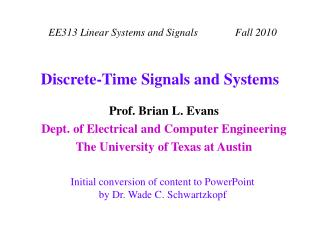Download PresentationDiscrete-Time Signals and Systems

# Discrete-Time Signals and Systems - PowerPoint PPT Presentation

Discrete-Time Signals and Systems. Signals. A function, e.g. sin( t ) in continuous-time or sin(2 p n / 10) in discrete-time, useful in analysis A sequence of numbers, e.g. {1,2,3,2,1} which is a sampled triangle function, useful in simulationI am the owner, or an agent authorized to act on behalf of the owner, of the copyrighted work described.
Download Presentation## Discrete-Time Signals and Systems

Download Policy: Content on the Website is provided to you AS IS for your information and personal use and may not be sold / licensed / shared on other websites without getting consent from its author.While downloading, if for some reason you are not able to download a presentation, the publisher may have deleted the file from their server.

- - - - - - - - - - - - - - - - - - - - - - - - - - E N D - - - - - - - - - - - - - - - - - - - - - - - - - -
Presentation Transcript
1. Discrete-Time Signals and Systems

2. Signals • A function, e.g. sin(t) in continuous-time orsin(2 p n / 10) in discrete-time, useful inanalysis • A sequence of numbers, e.g. {1,2,3,2,1} which is a sampled triangle function, useful insimulation • A collection of properties, e.g.even, causal, and stable, usefulinreasoningabout behavior • A piecewise representation, e.g. • A functional, e.g. d(t)

3. d[n] n Kronecker Impulse (Function) • Let d[n] be a discrete-time impulse function, a.k.a. the Kronecker delta function: • Impulse response h[n]: response of a discrete-time LTI system to a discrete impulse function 1

4. x(t) x[n] T{•} T{•} y(t) y[n] Systems • Systems operate on signals to produce new signals or new signal representations • Single-input one-dimensional continuous-time systems are commonly represented in two ways As operators As block diagrams

5. System Properties • Let x[n], x1[n], and x2[n] be inputs to a linear system and let y[n], y1[n], and y2[n] be their corresponding outputs • A linear system satisfies Additivity: x1[n] + x2[n]  y1[n] + y2[n] Homogeneity: a x[n]  a y[n] for any constant a • Let x[n] be the input to time-invariant system and y[n] be its corresponding output. Then,x[n - m]  y[n - m], for any integer m

6. s(t) Ts t Ts Sampled analog waveform Sampling • Many signals originate as continuous-time signals, e.g. conventional music or voice • By sampling a continuous-time signal at isolated, equally-spaced points in time, we obtain a sequence of numbers n {…, -2, -1, 0, 1, 2,…} Ts is the sampling period.

7. Fs = 44.1 kHz Ts = 0.023 ms Fs = 44.1 kHz Ts = 0.023 ms x(t) A/D OpticalDiskWriter OpticalDiskReader D/A x(t) v[n] CD v[n] Recording Studio Stereo System / PC Sampling • Consider audio compact discs (CDs) • Analog-to-digital (A/D) conversion consists of filtering, sampling, and quantization • Digital-to-analog (D/A) conversion consists of interpolation and filtering

8. n stem plot Generating Discrete-Time Signals • Uniformly sampling a continuous-time signal • Obtain x[n] = x(nTs) for - < n < . • How to choose Ts? • Using a formula • x[n] = n2 – 5n + 3, for n 0would give the samples{3, -1, -3, -3, -1, 3, ...} • We really do not know what the sequence looks like in continuous time because we do not have a sampling period associated with it